# 图片相似度计算及检索调研# 一 Hash 算法

Hash 算法作为大多图片搜索引擎的核心算法，其准确率和效率均很高，本板块将介绍 Hash 的三种核心算法：aHash、pHash、dHash。

## 1.1 图像均值哈希算法-aHash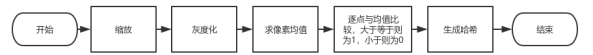1.缩放图片：为了保留结构去掉细节，去除大小、横纵比的差异，把图片统一缩放到 8*8，共 64 个像素的图片。

2.转化为灰度图：把缩放后的图片转化为 256 阶的灰度图。

3.计算平均值： 计算进行灰度处理后图片的所有像素点的平均值。

4.比较像素灰度值：遍历灰度图片每一个像素，如果大于平均值记录为 1，否则为 0.

5.得到信息指纹：组合 64 个 bit 位，顺序随意保持一致性即可。

6.对比指纹：计算两幅图片的指纹，计算汉明距离（从一个指纹到另一个指纹需要变几次），汉明距离越大则说明图片越不一致，反之，汉明距离越小则说明图片越相似，当距离为 0 时，说明完全相同。（通常认为距离>10 就是两张完全不同的图片）

## 1.2 图像感知哈希算法-pHash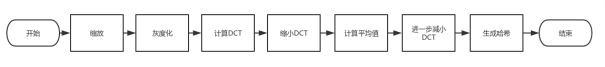1.缩小图片：32 * 32 是一个较好的大小，这样方便 DCT 计算

2.转化为灰度图：把缩放后的图片转化为 256 阶的灰度图。（具体算法见平均哈希算法步骤）

3.计算 DCT:DCT 把图片分离成分率的集合

4.缩小 DCT：DCT 是 32*32，保留左上角的 8*8，这些代表的图片的最低频率

5.计算平均值：计算缩小 DCT 后的所有像素点的平均值。

6.进一步减小 DCT：大于平均值记录为 1，反之记录为 0.

7.得到信息指纹：组合 64 个信息位，顺序随意保持一致性即可。

8.对比指纹：计算两幅图片的指纹，计算汉明距离（从一个指纹到另一个指纹需要变几次），汉明距离越大则说明图片越不一致，反之，汉明距离越小则说明图片越相似，当距离为 0 时，说明完全相同。（通常认为距离>10 就是两张完全不同的图片）

## 1.3 图像差异哈希算法-dHash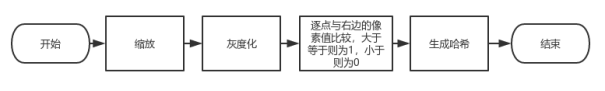1.缩小图片：收缩到 9*8 的大小，一遍它有 72 的像素点

2.转化为灰度图：把缩放后的图片转化为 256 阶的灰度图。（具体算法见平均哈希算法步骤）

3.计算差异值，获得最后哈希值（与 aHash 主要区别处）。dHash 算法工作在相邻像素之间。比较每行左右两个像素，如果左边的像素比右边的更亮（左边像素值大于右边像素值），则记录为 1，否则为 0。因为每行有 9 个像素，左右两个依次比较可得出 8 个值，所以 8 行像素共可以得出 64 个值，因此此时哈希值为长度是 64 的 0-1 序列。

4.图片配对，计算汉明距离。

## 汉明距离

1011101 与 1001001 之间的汉明距离是 2。

2143896 与 2233796 之间的汉明距离是 3。

"toned" 与 "roses" 之间的汉明距离是 3。

# 二 算法比较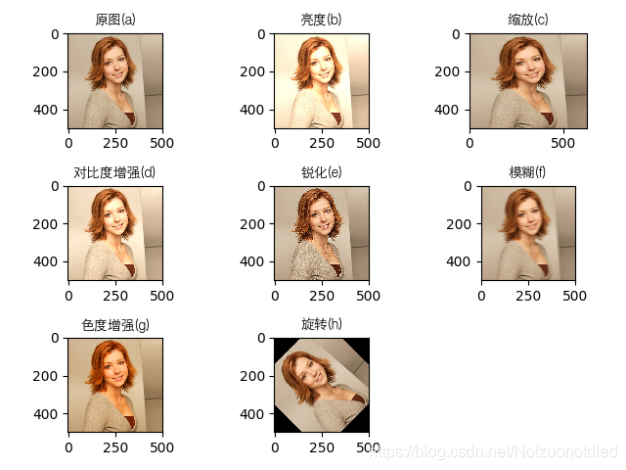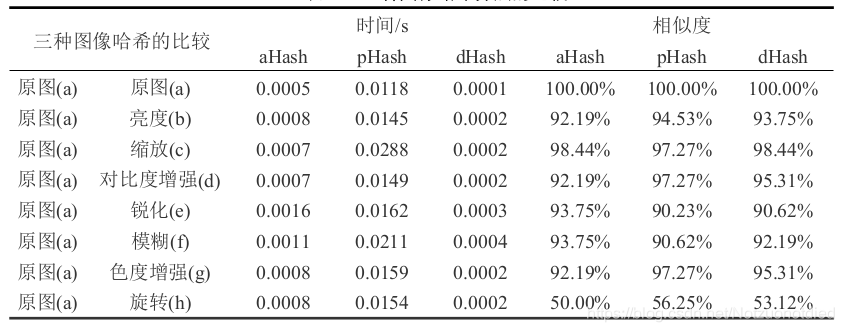# 三 环境搭建与算法实现

## 3.1 三种 hash 算法的实现与环境搭建

module 依赖：scikit-image ， opencv-python

sudo pip3 install --upgrade setuptoolssudo pip3 install scikit-image -i http://pypi.douban.com/simple/ --trusted-host pypi.douban.com//后面为镜像源加速pip install --default-timeout=1000 opencv-python//使用清华源安装pip3 install opencv-python -i https://pypi.tuna.tsinghua.edu.cn/simple

## 3.2 aHash、dHash、pHash 的实现示例

### 3.2.1 aHash

# -*- coding: utf-8 -*-from skimage import transformimport cv2#aHash方法计算图片的指纹数据img=cv2.imread('/Users/develop/imageanalysis/RPC框架.png')#读入图片length = 8height = 8sum = 0.0k = 0grey = []# 修改图像大小dst=transform.resize(img, (length, height))for i in range(length):    for j in range(height):        (b,g,r) = dst[i,j]        # 计算灰度值        grey.append(r*0.3+g*0.59+b*0.11)#浮点灰度值计算        sum+=grey[k]        k=k+1average = sum/(length*height)#算出平均的灰度数据fingerprint = []for i in range(length*height):    if grey[i] > average:        fingerprint.append(1)#如果灰度数据大于平均值，填入元素1，否则填入元素0    else:        fingerprint.append(0)print(fingerprint)

### 3.2.2 dHash

# -*- coding: utf-8 -*-from skimage import transform,dataimport matplotlib.pyplot as pltfrom skimage import ioimport cv2img=cv2.imread('/Users/develop/imageanalysis/RPC框架.png')#读入图片length = 9height = 8sum = 0.0k = 0grey = []dst=transform.resize(img, (length, height))#灰度图转化for i in range(length):    for j in range(height):        (b,g,r) = dst[i,j]        grey.append(r*0.3+g*0.59+b*0.11)#浮点灰度值计算        sum+=grey[k]        k=k+1# 获取每行的差值，为64位differ = []for i in range(length-1):#i 8    for j in range(height):#j 8        differ.append(grey[j*8+i+1]-grey[j*8+i])#获取01值fingerprint = []for i in range((length-1)*height):#8*8    if differ[i] > 0:        fingerprint.append(1)    else:        fingerprint.append(0)print(fingerprint)

### 3.2.3 pHash

# -*- coding: utf-8 -*-import cv2from skimage import transformimport numpy as np#利用phash计算图像指纹img=cv2.imread('/Users/develop/imageanalysis/RPC框架.png')#读入图片length = 32s_length = 8dst=transform.resize(img, (length, length))#print(type(dst))#all 代表全图，建议32*32matrix_all_grey = [[0 for i in range(length)] for i in range(length)]#small 代表最低频率，8*8大小matrix_small_grey = [[0 for i in range(s_length)] for i in range(s_length)]# 灰度图转化k = 0for i in range(length):    for j in range(length):        (b,g,r) = dst[i,j]        matrix_all_grey[i][j] = ( b + g + r )/3# 缩小fft_matrix_grey = np.fft.fft(matrix_all_grey)for i in range(s_length):    for j in range(s_length):        matrix_small_grey[i][j] = fft_matrix_grey[i][j]# 计算平均sum_pixel = 0for i in range(s_length):    for j in range(s_length):        sum_pixel += matrix_small_grey[i][j]average = sum_pixel / (s_length*s_length)bite = []#赋予01值for i in range(s_length):    for j in range(s_length):        if matrix_small_grey[i][j] > average :            bite.append(1)        else:            bite.append(0)print(bite)

## 3.3 高效对比检索

split_count = 8  # 每个64位的phash值分为八段，每段8位def split(key, split_count):    pre_length = 64 / split_count    return [key[i * pre_length: (i + 1) * pre_length] for i in range(split_count)]class ImageManager(object):    def __init__(self):        self.phash = pHash() # pHash类        self.phash_cache = [defaultdict(list) for i in range(split_count)] #        self.init_phash_map()    def init_phash_map(self):        #把所有的phash存在sqlite里边，这边取出所有的Image        for image in Image.select():            self.add_to_image_cache(image)    def add_to_image_cache(self, image):        # 将hash值分割为8段        key_split = split(bin(int(image.phash))[2:].rjust(64, '0'), split_count)        for index, k in enumerate(key_split):            self.phash_cache[index][k].append(image)    def has_same(self, ori_image):        phash = ori_image.phash        key_split = split(bin(int(phash))[2:].rjust(64, '0'), split_count)        result = set()        for index, k in enumerate(key_split):            if k in self.phash_cache[index]:                for image in self.phash_cache[index][k]:                    distance = self.distance(int(phash), int(image.phash))                    if distance < 5 and ori_image.key != image.key:                        result.add(image)        if result:            return True,list(result)        return False,[]

# 参考文章## 评论